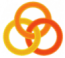General
 Course Home Page ESSLLI 2004Material
Lecture 1
 Slides Practical Session
Lecture 2
 Slides Practical Session
Lecture 3
 Slides Practical Session
Lecture 4
 Slides Practical Session
Lecture 5
 Slides Practical Session

# Solution

```%% An abcd string can consist of only a's and b's
abcd --> bc.

%% An abcd string can consist of an a followed by an abcd string
%% followed by a d
abcd --> onea, abcd, oned.

%% A bc string can be empty.
bc --> [].

%% Otherwise, it consists of two b's followed by a bc string followed
%% by two c's.
bc --> oneb, oneb, bc, onec, onec.

onea --> [a].
oneb --> [b].
onec --> [c].
oned --> [d].
```